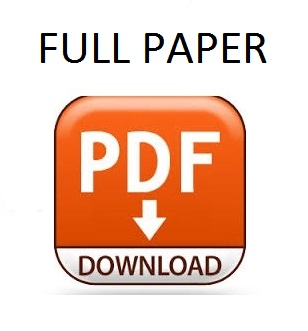# Wireless Power Transfer Analysis Using Scattering Parameters

### Abstract

 Wireless power transfer systems (WPTS) can be made more efficient from the emitter-receiver and from the active power transfer efficiency point of view by using the scattering parameters S. Using electrical circuit theory and starting from the correct formulation of scattering parame-ters, S parameters are computed in an automated manner based on modified nodal equations corresponding to WPTS. Advanced computation software (ADS, Ansoft Extractor Q3D, Cadence, etc.) is used to compute the parameters cor-responding to the magnetically coupled coils. The magneti-cally coupled coils can be described by the parameters of the resulting equivalent circuit, such as: impedance and admit-tance matrices, transfer coefficients matrix and the scatter-ing parameters matrix. This paper presents on one hand the correct formulation of S parameters and on the other hand the practical use of these parameters to streamline the wire-less energy transfer. To generate the analyzed parameters, dedicated function were developed in MATLAB and exist-ing routines from the Microwave toolbox (MATLAB) have been used. The frequency variation of S has been studied using graphical representation and Smith diagram. The accuracy in defining the scattering parameters S, based on analog circuit theory in harmonic state, in complex domain, is confirmed by the results obtained using the program ADS, that contains routines specific for generating S parameters.